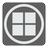Counting Tiles
Elementary+
English ES JA RUStephan needs some help building a circular landing zone using the ice square tiles for their new Ice Base. Before he converts the area to a construction place, Stephan needs to figure out how many square tiles he will need.

Each square tile has size of 1x1 meters. You need to calculate how many whole and partial tiles are needed for a circle with a radius of N meters. The center of the circle will be at the intersection of four tiles. For example: a circle with a radius of 2 metres requires 4 whole tiles and 12 partial tiles.Input: The radius of a circle as a float

Output: The quantities whole and partial tiles as a list with two integers -- [solid, partial].

Example:
```checkio(2) == [4, 12]
checkio(3) == [16, 20]
checkio(2.1) == [4, 20]
checkio(2.5) == [12, 20]
```

How it is used: This task is a simple geometry problem; but you can use it for architecture and topography. As we see in the description, you can calculate the amount of materials needed for a project.

Precondition: